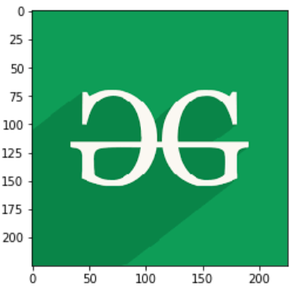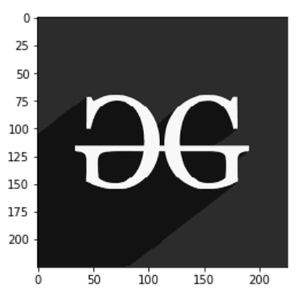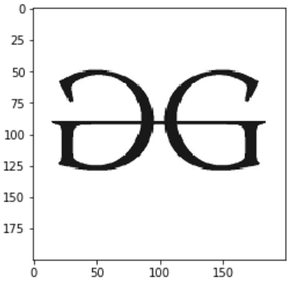How to Display an Image in Grayscale in Matplotlib?

• Difficulty Level : Easy
• Last Updated : 12 Dec, 2021

In this article, we are going to depict images using matplotlib module in grayscale representation i.e. image representation using two colors only i.e. black and white.

Required modules

• PIL is the Python Imaging Library which provides the python interpreter with image editing capabilities. The Image module provides a class with the same name which is used to represent a PIL image. The module also provides a number of factory functions, including functions to load images from files, and to create new images. PIL.Image.open() method in PIL module opens and identifies the given image file.
• Matplotlib is a plotting library for creating static, animated, and interactive visualizations in Python. The matplotlib module can be used in Python scripts, the Python and IPython shell, web application servers, and various graphical user interface toolkits like Tkinter, awxPython, etc.

Step-by-step Approach:

• Import required modules

Python3

 # importing libraries.import numpy as npimport matplotlib.pyplot as pltfrom PIL import Image
• Displaying Original picture.

Python3

 # storing image pathfname = r'g4g.png' # opening image using pilimage = Image.open(fname) # plottingimageplt.imshow(image)plt.show()

Output:• Displaying Grayscale image, store the image path here let’s say it fname. Now open the image using PIL image method and convert it to L mode If you have an L mode image, that means it is a single-channel image – normally interpreted as grayscale. It only stores a grayscale, not color. Plotting the image as cmap = ‘gray’ convert the colours. All the work is done you can now see your image.

Python3

 # storing image pathfname = r'g4g.png' # opening image using pilimage = Image.open(fname).convert("L") # maping image to gray scaleplt.imshow(image, cmap='gray')plt.show()

Output:Below are some programs which depict how to display an image in grayscale using Matplotlib module:

Example 1:

Image used:Python3

 # importing libraries.import numpy as npimport matplotlib.pyplot as pltfrom PIL import Image  # storing image pathfname = r'gfg.png' # opening image using pilimage = Image.open(fname).convert("L") # maping image to gray scaleplt.imshow(image, cmap='gray')plt.show()

Output:Example 2:

Image Used:Python3

 # importing libraries.import numpy as npimport matplotlib.pyplot as pltfrom PIL import Image  # storing image pathfname = r'geeks.png' # opening image using pilimage = Image.open(fname).convert("L") # maping image to gray scaleplt.imshow(image, cmap='gray')plt.show()

Output:My Personal Notes arrow_drop_up